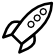﻿ Rocket Thrust Tool

# Thrust CalculatorThis macro tool was written to simplify the multiple step process of calculating the thrust through a clip plane at the exit of a nozzle.

The intention is that a user selects a plane (created or read in), which we'd like to calculate the thrust through, based on the following generalization:

Thrust = (mdot*Ve) + (Pe - Pinf)*Area

There are two methods which are utilized to calculate this Thrust : Elemental Distribution (Method 1), or Average (Method 2)

Method 1: Based on elemental distribution on the plane, where:

mdot = mass flow rate through each element (scalar)

Ve = velocity in the thrust direction for each element (scalar)

Pe = pressure for each element (scalar)

Pinf = reference Pressure (constant)

Area = Elemental size for each element (scalar)

Here, the individual Thrust through each element on the plane is calculated first, then summed over all of those elements, yielding this equation:

Thrust = SUM[(mdot*Ve) + (Pe - Pinf)*Area]

Method 2: Based on Averages on the Clip plane. This uses the same equation:

Thrust = (mdot*Ve)+(Pe - Pinf)*Area

Where:

mdot = total mass flow rate through the plane.

Ve = average velocity across the plane (in the direction parallel to the thrust direction)

Pe = average Pressure (absolute) on the plane.

Pinf = reference Pressure

Area = Area of the plane.

Method 2 essentially works off from the averages on the plane, and then approximates the thrust based on these averages.

If there is no/little variation across the plane, the two methods should return exact/similar values. If the variation across the plane is large, these two methods will yield different results (typically 2-5% different), with method 1 respecting the spatial variation.